# Lecture 20: Black-box optimization and reinforcement learning¶

In this lecture, we'll look at derivative-free optimization and reinforcement learning. Starting with a quick dive into derivative-free optimization, we'll see that the concepts we learn there naturally connect with reinforcement learning. Along the way, we learn how to solve the popular Flappy Bird game!

Overview:

Acknowledgements: Many thanks to Ben Recht for suggestions based on his recent blog post series. The second part of this lecture is mostly comes from his blog posts (with the exception of flappy bird).

In :
%matplotlib inline

import scipy.optimize
import numpy as np
import matplotlib.pyplot as plt
from mpl_toolkits.mplot3d import Axes3D
from plotters import error_plot, kwargs, setup_layout

np.random.seed(1)
setup_layout()


## Black-box optimization¶

In this lecture we will study what is known as black-box optimization, derivative-free optimization, or zeroth-order optimization.

Here, the general goal is to solve a problem

$$\min_{x} f(x),$$

possibly over a constraint set $x\in\Omega,$ where we do not have access to the gradients of the function $f\colon\mathbb{R}^n\to\mathbb{R}$. Rather we can only query points $x\in\Omega$ and receive the function evaluation $f(x)\in\mathbb{R}.$

Random search

Pick an initial point $x_0$

Iterate from $t=1$:

• Pick $d_t$ be a random direction in $\mathbb{R}^n.$
• Set $\eta^* = \arg\min_\eta f(x_{t-1}+\eta d_t)$
• Put $x_t = x_{t-1} + \eta^* d_t$

#### Analysis¶

We'll see that essentially whatever was true for gradient descent is also true for this algorithm at the cost of a factor $n$ in the number of iterations. To see the idea, assume that $f$ is a $\beta$-smooth convex, differentiable function.

For any $\eta,$ by Taylor's theorem and smoothness, we have:

$$f(x_t + \eta d_t) \le f(x_t) + \eta \langle d_t, \nabla f(x_t) \rangle + \frac{\eta^2\beta}{2}\|d_t\|^2$$

And hence, minimizing the upper bound, there is a step size $\eta$ for which we have

$$f(x_t + \eta d_t) \le f(x_t) - \frac{1}{\beta}\langle d_t, \nabla f(x_t)\rangle^2 \|d_t\|^2$$

Moreover, the step size we actually took can only be better:

$$f(x_t + \eta^* d_t) \le f(x_t + \eta d_t).$$

Taking expectations then shows

$$\mathbb{E}[f(x_t + \eta^* d_t)] \le \mathbb{E}[f(x_t)] - \frac1{\beta n}\mathbb{E}[\|\nabla f(x_t)\|^2]$$

This looks just like what we know for gradient descent with an extra factor of $n$. Now we can use whatever assumptions we want:

• For convex functions, we get a rate of $n\beta/\epsilon$.
• For strongly convex, you get $n\beta\log(1/\epsilon)$.
• For non-convex functions, we converge to a stationary point.

Basically, it's $n$ times the complexity of the gradient method on the function class.

#### Line search subproblem¶

As stated the algorithm uses an optimal step size in a random direction. We can generally only approximate this step in various ways. We'll discuss what's known as Golden-Section Search:

In :
# Source: https://en.wikipedia.org/wiki/Golden-section_search

def gss(f, a, b, tol=1e-5):
"""Golden section search

Find the minimum of f on [a,b]
f: a strictly unimodal function on [a,b]
"""
gr = (np.sqrt(5) + 1) / 2
c = b - (b - a) / gr
d = a + (b - a) / gr
while abs(c - d) > tol:
if f(c) < f(d):
b = d
else:
a = c
# we recompute both c and d here to avoid loss of precision which may lead to incorrect results or infinite loop
c = b - (b - a) / gr
d = a + (b - a) / gr
return (b + a) / 2


With line search in place we can code up our random search algorithm.

In :
def random_search(oracle, init, num_steps, line_search=gss):
"""Implements random search.

oracle: Function.
init: Point in domain of oracle.
num_steps: Number of iterations.
line_search: Line search method (defaults to golden section.)

Returns: List of iterates."""
iterates = [init]
for _ in range(num_steps):
d = np.random.normal(0, 100, init.shape)
d /= np.linalg.norm(d)
x = iterates[-1]
eta = line_search(lambda step: oracle(x + step * d), -1, 1)
iterates.append(x + eta * d)
return iterates


Let's sanity check this on a least squares problem.

In :
A = np.random.normal(0, 1, (100, 100))
b = np.random.normal(0, 1, (100,))
def least_squares(x):
return 0.5*np.linalg.norm(A.dot(x)-b)**2

In :
xs = random_search(least_squares, b, 1000, gss)
error_plot([least_squares(x) for x in xs])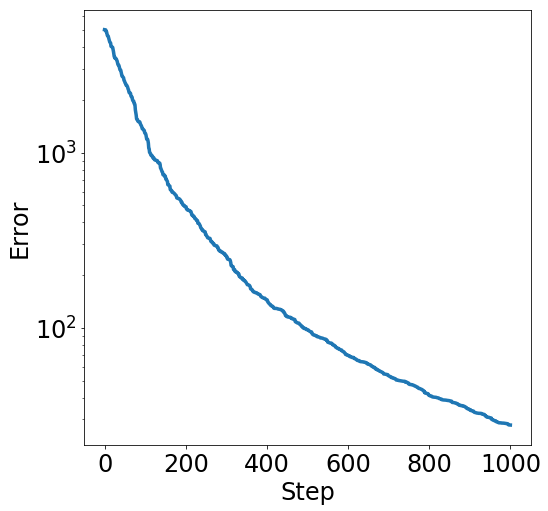Nelder and Mead proposed a more sophisticated variant of random search.

In :
def nelder_mead(oracle, init, num_steps):
"""Wrapper around scipy Nelder-Mead search algorithm."""
iterates = [init]
scipy.optimize.minimize(oracle, init,
callback=lambda x: iterates.append(x),
options={'maxiter' : num_steps},
return iterates


Let's try this out on a common non-convex test function.

In :
def himmelblau(x):
"""Himmelblau's function."""
return (x**2 + x - 11)**2 + (x + x**2 -7)**2

In :
points = np.linspace(-5, 5, 1000)
X, Y = np.meshgrid(points, points)
fig = plt.figure(figsize=(15,15))
ax = fig.gca(projection='3d')
ax.plot_surface(X, Y, himmelblau([X, Y]), cmap=plt.cm.coolwarm);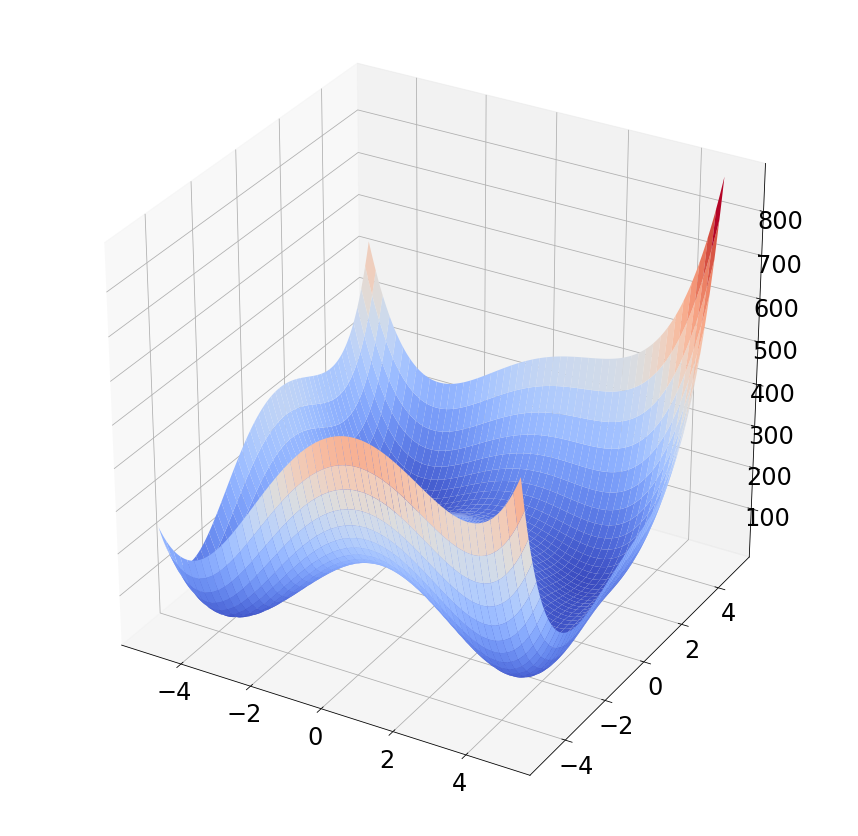In :
xs = nelder_mead(himmelblau, [1,1], 50)
error_plot([himmelblau(x) for x in xs])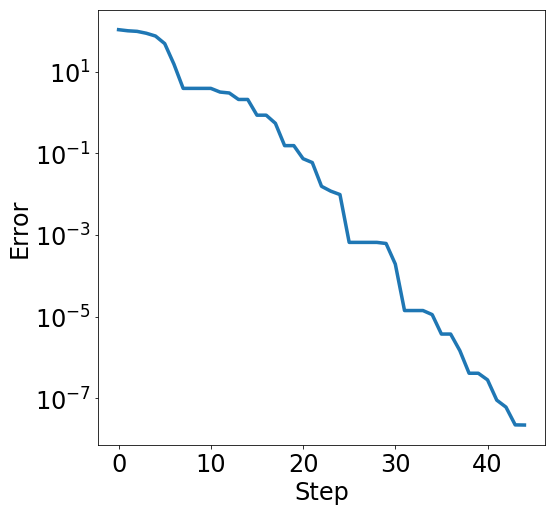Random search actually turns out to also do pretty well here.

In :
xs = random_search(himmelblau, np.array([1,1]), 50)
error_plot([himmelblau(x) for x in xs])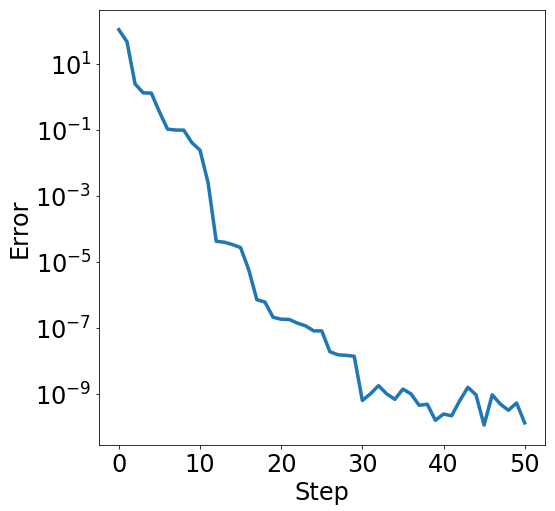## Reinforcement learning in 45 minutes¶

Consider an abstract dynamical system model

$$x_{t+1} = f( x_t, u_t, e_t)\,.$$

Here, $x_t$ is the state of the system, $u_t$ is the control action, and $e_t$ is a random disturbance. We’re going to assume that $f$ is fixed, but unknown.

A trajectory is a sequence of states and control actions generated by this dynamical system.

$$\tau_t = (u_1,…,u_{t-1},x_0,…,x_t) \,.$$

A control policy (or simply “a policy”) is a function, $\pi$, that takes a trajectory from a dynamical system and outputs a new control action. Note that $\pi$ only gets access to previous states and control actions.

### Flappy bird dynamical system¶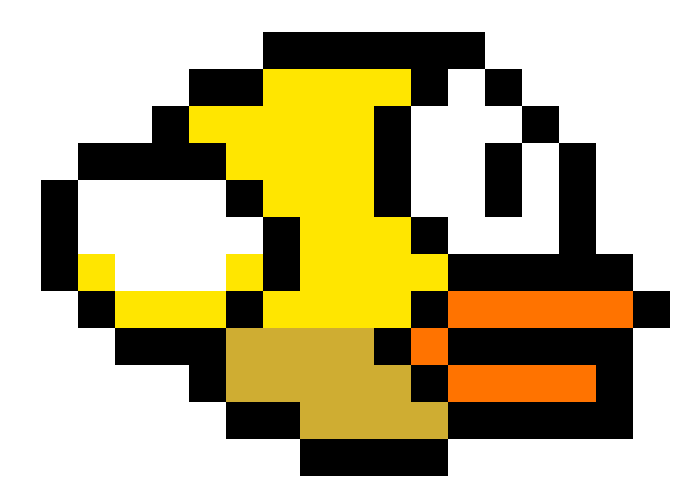Image source

Imagine we have a little bird flying in the air at constant velocity. Its vertical position $h$ is governed by gravity and air resistance. So, the total downward force is $F = mg - kv,$ where $g$ is the acceleration due to gravity, $v$ is the downward velocity and $k$ is a drag coefficient that relates air resistance to velocity. Using $F=ma,$ this gives the first-order differential equation $\frac{dv}{dt}= g - (k/m)v.$

The bird can choose to accelerate upwards. It's trying to avoid hitting the ground $h=0,$ while minimizing upward acceleration.

In :
def flappy_bird(state, action):
"""Flappy bird dynamical system.

state: pair of downward velocity and height
action: upward acceleration

Returns new state."""
v, h = state
g = 1. # acceleration due to gravity
k = 0.01 # drag coefficient
m = 1 # mass
return (v + g - (k/m)*v - action, h - v)

def unroll(system, inits, policy, num_steps):
"""Unroll a dynamical system."""
states = [inits]
actions = [inits]
for _ in range(num_steps):
action = policy(states, actions)
actions.append(action)
states.append(system(states[-1], action))
return states, actions

def example_policy(states, actions):
"""Accelerate upwards every 5 steps."""
v, h = states[-1]
if divmod(len(states), 5) == 0:
return 7
else:
return 0.

flappy_inits = [(0, 10.), 0]
states, actions = unroll(flappy_bird, flappy_inits, example_policy, 10)
heights = [h for (v, h) in states]

plt.xkcd(); fig = plt.figure(figsize=(15,10))
fig.gca().imshow(im, aspect='auto', extent=(0, 1, 9.5, 10.5))
fig.figimage(im, 0, fig.bbox.ymax)
plt.ylabel('height'); plt.xlabel('time');
plt.plot(range(len(heights)), heights, **kwargs);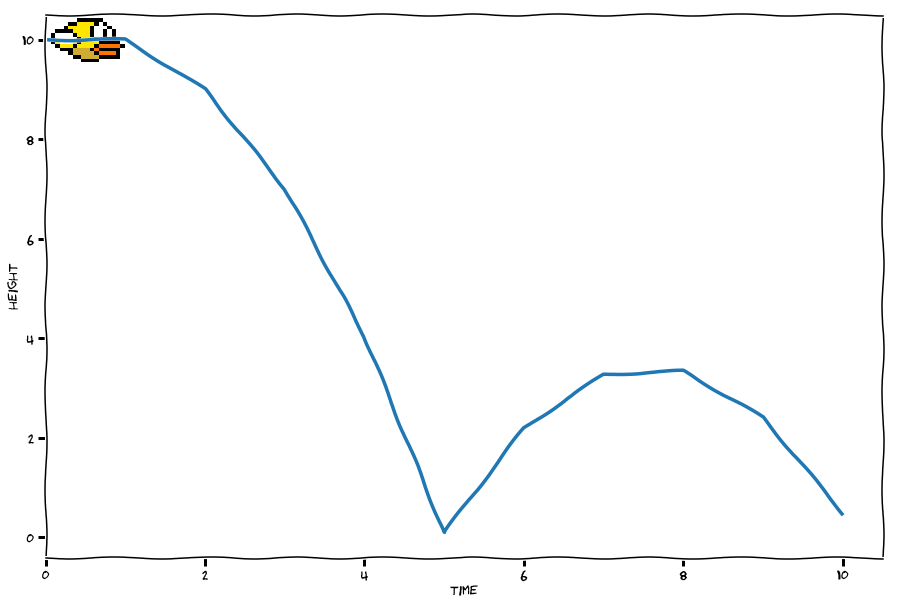Our policy looks good on a short horizon, but in the long term it goes way higher than it needs to.

In :
states, actions = unroll(flappy_bird, flappy_inits, example_policy, 20)
heights = [h for (v, h) in states]
plt.figure(figsize=(15,5))
plt.ylabel('height'); plt.xlabel('time')
plt.plot(range(len(heights)), heights, **kwargs);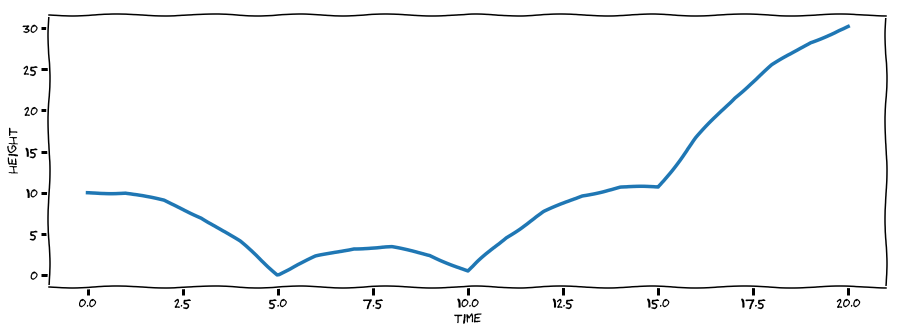At this point, we could either use physics (but that's too hard for me!) or try to learn a good policy!

### Learning policies from rewards¶

The main goal in reinforcement learning is to solve the optimal control problem:

$$\begin{array}{ll} \mbox{maximize}_{u_t} & \mathbb{E}_{e_t}[ \sum_{t=0}^N R_t[x_t,u_t] ]\\ \mbox{subject to} & x_{t+1} = f(x_t, u_t, e_t)\\ & \mbox{(x_0 given)} \end{array}$$

• $R_t[x_t,u_t]$ is a reward, which we assume is known.
• The function $f$ is unknown.

In our flappy bird example, we'd get a huge negative reward for hitting the ground, and a small negative reward for accelerating upwards.

The main paradigm in contemporary RL is the following approach. We decide on a policy $\pi$ and horizon length $L$. Then we either pass this policy to a simulation engine or to a real robotic system and are returned a trajectory

$$\tau_L = (u_1,…,u_{L-1},x_0,…,x_L)\,,$$

where $u_t = \pi(\tau_t)$. This is our oracle model. We typically want to minimize the total number of samples computed by the oracle. So if we were to run $m$ queries with horizon length $L$, we would pay a total cost of $mL$. However, we are free to vary our horizon length for each experiment.

In the policy gradient approach, we imagine that policies are randomized and specified be a set of parameters $\theta$ that we can tune.

We can then write the reward maximization problem as a general stochastic optimization problem of the form. Let $p_\theta$ denote the probability distribution over trajectories resulting from our the parameters $\theta.$ Below we use $\mathbb{E}_\theta$ to denote the expectation over trajectories drawn from $p_\theta$.

$$J(\theta):=\mathbb{E}_\theta[R(u)]$$

The key idea is to compute the gradient of $J(\theta)$ using the following trick.

\begin{align*} \nabla_{\theta} J(\theta) &= \int R(u) \nabla_{\theta} p_\theta(u) \,\mathrm{d}u\\ &= \int R(u) \left(\frac{\nabla_{\theta} p_\theta(u)}{p_\theta(u)}\right) p_\theta(u) \,\mathrm{d}u\\ &= \int \left( R(u) \nabla_{\theta} \log p_\theta(u) \right) p_\theta(u)\,\mathrm{d}u \\ &= \mathbb{E}_{\theta}\left[ R(u) \nabla_{\theta} \log p_\theta(u) \right]\,. \end{align*}

And hence the following is a general purpose algorithm for maximizing rewards with respect to parametric distributions:

Choose some initial guess $\theta_0$

REINFORCE:

Iterate for $t\ge 1:$

• Sample $u_k$ i.i.d., from $p_{\theta_k}$.
• Set $\theta_{k+1} = \theta_k + \alpha_k R(u_k) \nabla_{\theta} \log p_{\theta_k}(u_k)$, where $\alpha_k$ is some step size.

Note that the reward function we want to optimize is only accessed through function evaluations. We never compute gradients of $R$ itself. Therefore, this method is best thought of as a zeroth-order optimization algorithm akin to random search or Nelder-Mead.

The original source of this section is this blog post. Check it out as it has additional details.

### "Solving" flappy bird¶

Let's see how these ideas can get us a good policy for the flappy bird game.

In :
def flappy_reward(states, actions):
"""Rewards for flappy bird game."""
reward = 0.0
for (state, action) in zip(states, actions):
_, height = state
if height < 0:
reward += 10 * height
reward -= action
return reward

def flappy_policy(theta, states, actions):
"""Linear map of last two states, ignoring previous actions."""
if len(states) == 1:
states = states + states
return np.dot(theta, np.reshape(np.array(states[-2:]), (4,)))

def flappy_simulator(theta, num_steps):
"""Compute rewards of policy for given number of steps."""
policy = lambda sts, ats: flappy_policy(theta, sts, ats)
states, actions = unroll(flappy_bird, flappy_inits, policy,
num_steps)
return flappy_reward(states, actions)


Since we just convinced ourselves that policy gradient is essentially zero-order optimization of the reward function, let's just use our random search algorithm from before to find a good policy. This is even simpler than REINFORCE, since we don't need to decide on a distribution over our policy family.

In :
def objective(theta, horizon=20):
"""Flappy bird search objective."""
return -1.0*flappy_simulator(theta, horizon)

In :
xs = random_search(objective, np.zeros(4), 10, gss)
policy = lambda states, actions: flappy_policy(xs[-1], states, actions)
states, actions = unroll(flappy_bird, flappy_inits, policy, 20)
heights = [h for (v, h) in states]

fig = plt.figure(figsize=(15, 10))
fig.gca().imshow(im, aspect='auto', extent=(0, 1, 9.5, 10.5))
fig.figimage(im, 0, fig.bbox.ymax)
plt.plot(range(len(heights)), heights, **kwargs);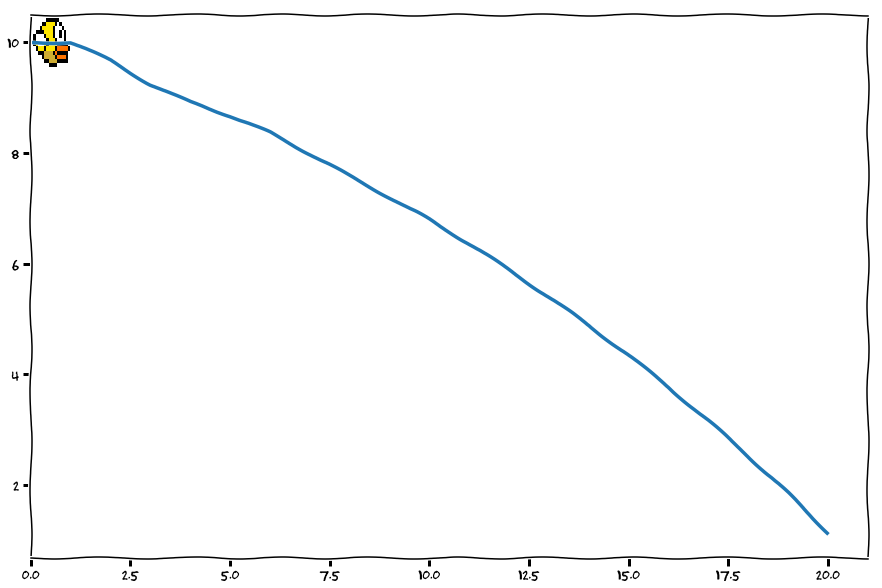We see that the bird avoids ever hitting the ground on the horizon it was trained for, while minimizing the amount of upward acceleration necessary.

Exercise: Re-run this code a few times to see that the results vary greatly with random seed! This issue leads us to the next section.

### Sample complexity considerations¶

Let's discuss some sample complexity issues taken from this blog post by Ben Recht.

Consider a simple objective that arises from the Linear Quadratic Regulator (LQR) problem in control theory:

$$R(u) = -||u-z||^2$$

Let $p_\theta$ be a multivariate Gaussian with mean $\theta$ and covariance matrix $\sigma^2 I$. What does policy gradient do? First, note that

$$J(\theta) = \mathbb{E}_{\theta} [R(u)]= -\|\theta-z\|^2 - \sigma^2 d$$

As a function of $\theta$, $J$ is strongly concave, and the most important quantity to understand the convergence of policy gradient is the expected norm of the gradient as this will control the number of iterations. Now, if you start at $\theta=0$, then the gradient is

$$g=\frac{||\omega-z||^2 \omega}{\sigma^2}\,,$$

where $\omega$ is a normally distributed random vector with mean zero and covariance $\sigma^2 I$. If you grind through the details, you'll see the expected norm of this gradient is on the order of

$$O\left(\sigma d^{1.5} + \sigma^{-1} d^{0.5} \|z\|\right)\,.$$

That's quite large in the dimension of the problem. For a better way to solve LQR and other problems, check out this post.